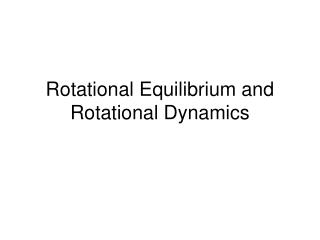DownloadDownload PresentationRotational Equilibrium and Rotational Dynamics

# Rotational Equilibrium and Rotational Dynamics

Télécharger la présentation## Rotational Equilibrium and Rotational Dynamics

- - - - - - - - - - - - - - - - - - - - - - - - - - - E N D - - - - - - - - - - - - - - - - - - - - - - - - - - -
##### Presentation Transcript

1. Rotational Equilibrium and Rotational Dynamics

2. Read introduction page 226 • If F is the force acting on an object, and r is position vector from a chosen point O to the point of application of the force, with F perpendicular to r. The magnitude of the TORQUE σ exerted by the force F is: τ = r F SI unit : Newton-meter (Nm)

3. When an applied force causes an object to rotate clockwise, the torque is positive • When the forces causes an objet to rotate counterclockwise, the torque of the object is negative • When two or more torques act on an object at rest the torques are added • The rate of rotation of an object doesn’t change, unless the object is acted on by a torque

4. The magnitude of the torque τ exerted by the force F is: τ = F r sinθ Where r- F- θ- The value of τdepends on the chosen axis of rotation

5. The direction of σ is given by the right-hand-rule • An object in mechanical equilibrium must satisfy: 1. The net external forces must be zero: ΣF = 0 2. The net external torque must be zero: Στ = 0

6. We wish to locate the point of application of the single force of magnitude w=Fg where the effect on the rotation of the object is the same as that of the individual particles – center of gravity (m1g+m2g+..mng)xcg= m1gx1+m2gx2+…mn g xn xcg=Σmixi / Σmi ycg=Σmiyi / Σmi zcg=Σmizi / Σmi

7. Problem solving strategy for objects in equilibrium • Diagram system • Draw the free body diagram • Apply Στ = 0, the second condition of equilibrium • Apply ΣF = 0 (on x axis and y axis) • Solve the system of ecuation

8. Relationship between torque and angular acceleration: Ft = mat. Ft r = mat r at = r α Ft r = m r2α τ = m r2α m r2is called momentum of inertia

9. Torque on a rotational object: Στ =(Σm r2)α Σm r2= m1r12+m2r22+… The momentum of inertia of the whole body: I= Σm r2 Στ = I α = I α The angular acceleration of an extended rigid object is proportional to the net torque acting on it

10. M = m1+m2+… I= Σm r2= m1r12+m2r22+… I = (m1+m2+…) R2 I = MR2

11. An object roatating about some axis with an angular speed ω has rotational kinetic energy: ½ I ω2. v = r ω KEτ= Σ(½ m v2) = Σ(½ mr2ω2) = Σ(½ mr2 )ω2 =½ I ω2.

12. Conservation of mechanical energy: (Ex. a bowling ball rolling down the ramp) (KEt + KEτ +PE)i = (KEt + KEτ +PE)f KEt – translational KE KEτ– rotational KE PE – gravitational potential energy Work –Energy of mechanical energy: Wnc = ΔKEt + Δ KEτ + Δ PE

13. Problem solving strategy (energy and rotation) • Choose two points of interest • Identify conservative and nonconservative forces • Write the work energy theorem • Substitute general expression • Use v = r ω • Solve the unknown

14. Angular momentum: An object of mass m roatates in an circular path of radius r, acted by a net force F, resulting a net torque τ Στ= Iα = I (Δω/Δt) = I(ω –ω0) /Δt = (Iω –Iω0) /Δt Angular momentum: L = Iω Στ=ΔL /Δt = change in angular momentum / time interval If Στ= 0, angular momentum is conserved : Li =Lf